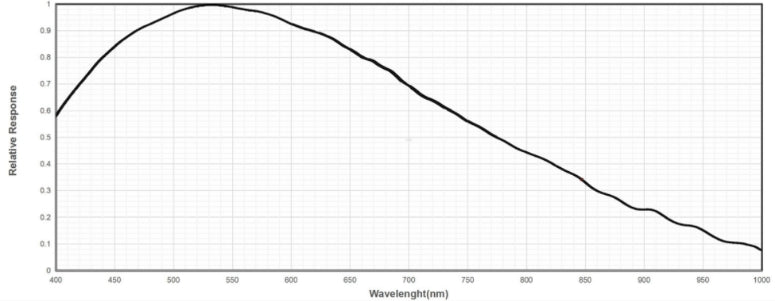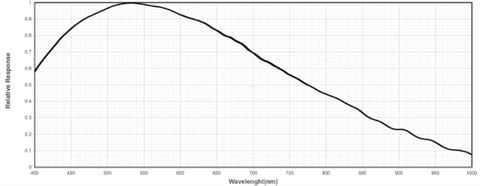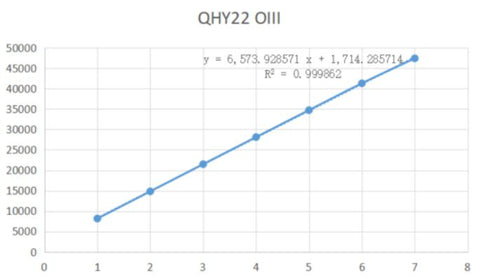# Measuring the Absolute QE of the QHY268M

Sony provides relative QE curves for the IMX571 sensor used in the QHY268M camera.  However, by comparing its response with a CCD of known absolute QE, the ICX694 used in the QHY22, we can determine the absolute QE for the QHY268M.

The graph below from the Sony IMX571 datasheet shows the relative QE of the IMX571.The good news here is that this curve includes the near infrared wavelengths.  The QHY600M graph, for example, only shows thed  QE in the range of 400 – 700nm.  However, for the QHY268M we have the curve from 400 – 1000nm.  It is good to know the near infrared response from 700 – 1000nm.Sony’s official curves for QE are good for showing the relative response of the sensor across a range of wavelengths but, typical of Sony’s QE data, the peak QE is always equal to 1 or 100%.  In order to get the absolute QE, key information for astronomical and scientific imaging, we performed the following experiment to find it.

First, we need a sensor with known QE.  In this case we used the well-known Sony ICX694. This sensor, used in many popular CCD cameras like the QHY22, ATIK460, QSI and others, has been characterized by others like Christian Buil.  The QE curve can be found here:

http://www.astrosurf.com/buil/cameras/index.html (ref.1).

Also, there is another value at

http://www.astrosurf.com/buil/CMOSvsCCD/index.html  (ref.2).

To find a fit for the curve, we select one wavelengths for measurement.  In this case we use an OIII narrow band filter to measure the response at the wavelengths of 500 to 505nm, because this point is very close to the peak QE point and the error will be smallest.  From the ICX694 QE data we know that at OIII the absolute QE is 67% (ref.1) or 63% (ref.2).

Using the same light source and lens, we measured image intensity at different exposure times for both a QHY22 and a QHY268M.  Then we derive a curve of the intensity increasing with exposure time.  With linear fitting we get the number of ADU’s per second in both cameras.The system gain of the QHY268M on the gain/readmode of this experienment is 0.36e/ADU and the pixel area is 14.13 um^2.  For the QHY22 the system gain is 0.34e/ADU and the pixel area is 20.25um^2.

 IntensityADU/sec/px Intensitye/sec/um^2 QHY268M OIII 6036 153.78 QHY22 OIII 6574 110.36

From above table we can see that at the wavelength OIII it is 1.39 times that of the QHY22.

 ICX694 RATIO IMX571 63% (ref 2) 1.39 87.57% 67% (ref 1) 1.39 93.13%

From the Sony data we know the relative QE value is 0.97 at OIII.  Therefore, the peak QE for the IMX571 is calculated as 0.8757*(1/0.97)*100%= 90.2% or 0.9313*(1/0.97)*100% = 95.9%.

Conclusion
The test results shows that the QE at OIII is at least 87% and the peak QE of QHY268M is at least 90%.  As expected, this test result is very similar to the results obtained for the QHY600M (QHY600 results is 87% @ OIII).

Based on the above tests, we have derived the absolute QE curve of QHY268M from 400nm-1000nm seen here: